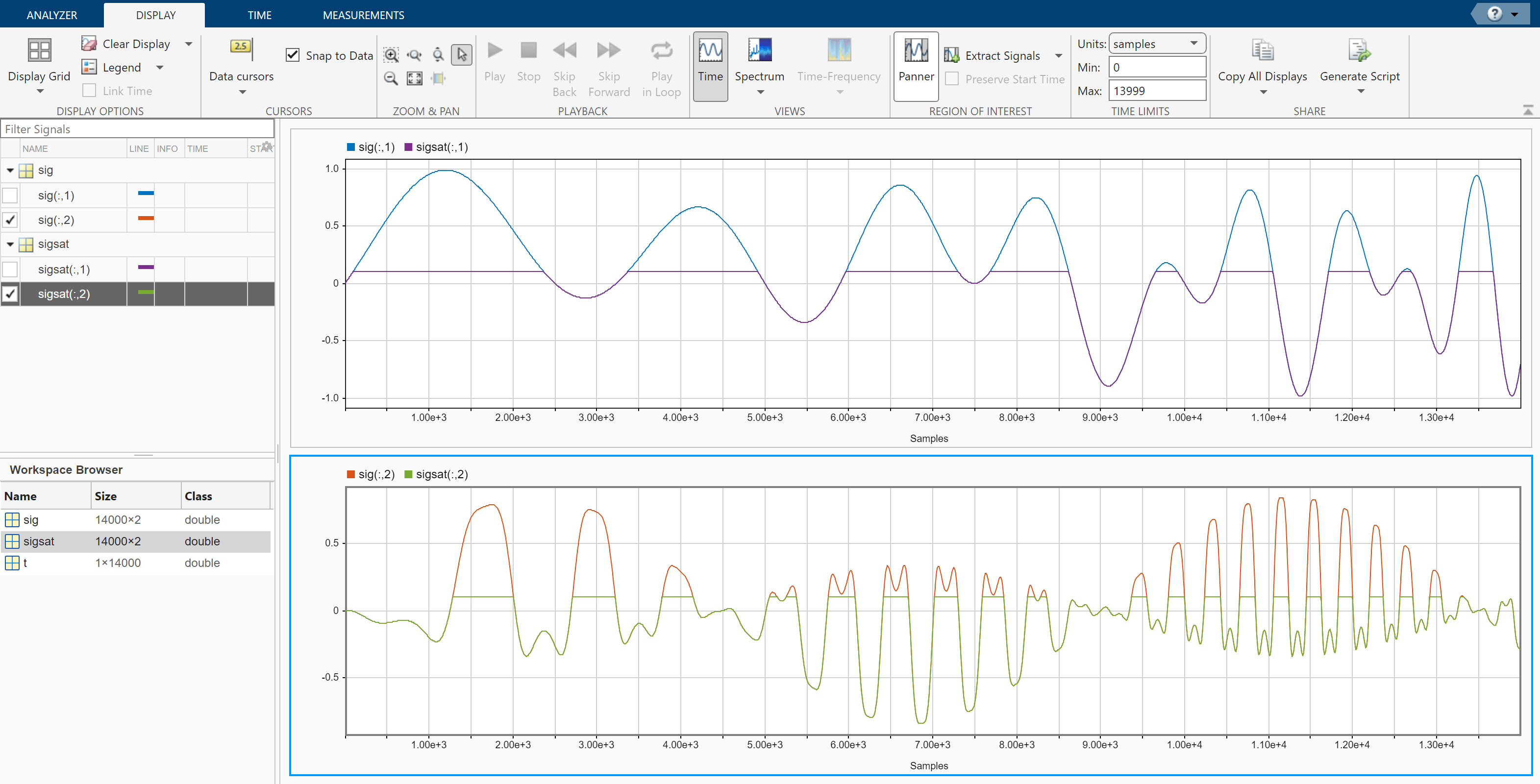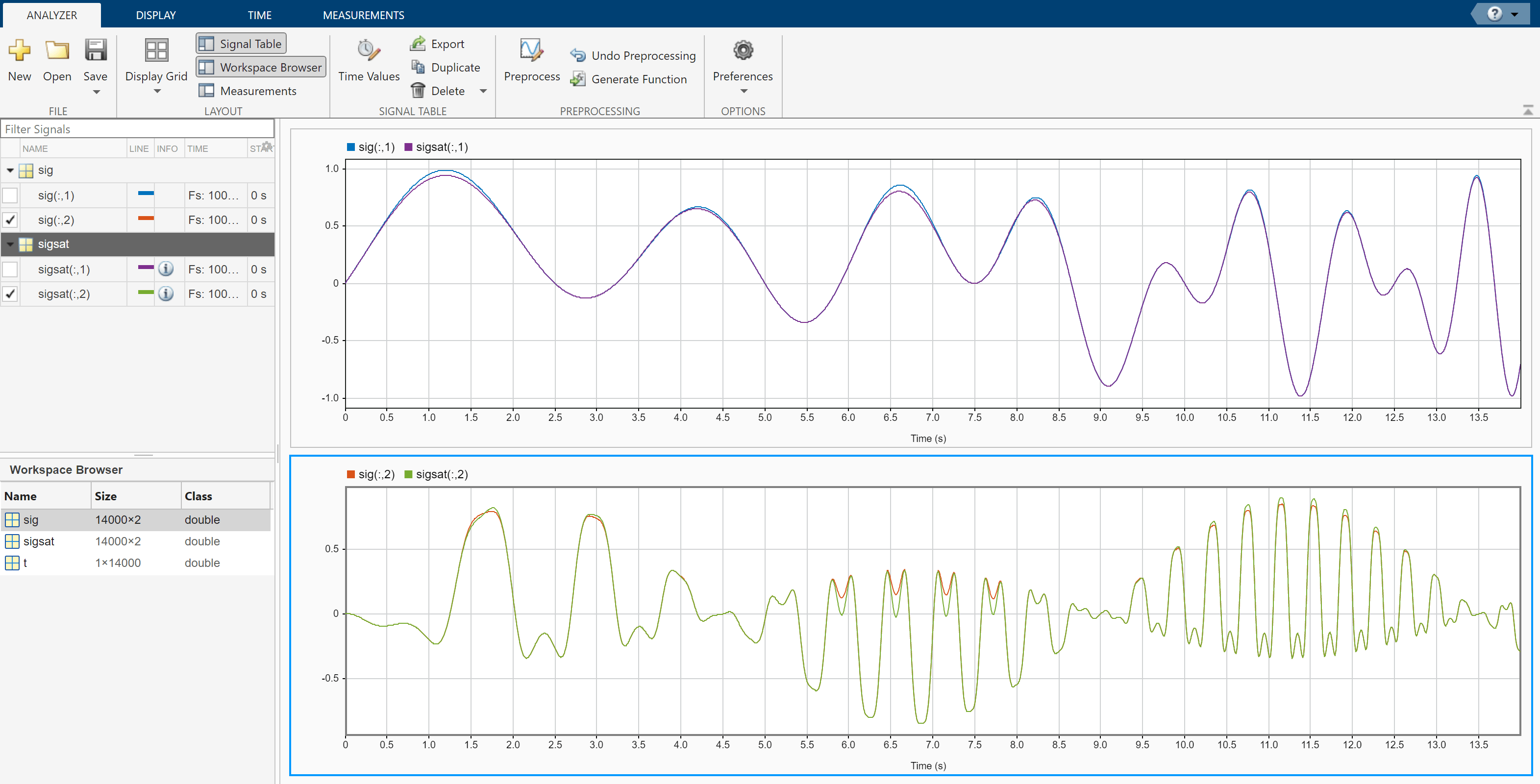Declip Saturated Signals Using Your Own Function

Sensors can return clipped readings if the data are larger than a given saturation point. To reconstruct the readings, you can fit a polynomial through the points adjacent to the saturated intervals. Write a function that performs the reconstruction and integrate it into Signal Analyzer.

Generate a three-channel signal sampled at 1 kHz for 14 seconds. The signal has several peaks of varying sizes and shapes. A sensor that reads the signal saturates at 0.1 V.

fs = 1000;
t = 0:1/fs:14-1/fs;

0.85*besselj(0,5*(sin(2*pi*(t+1.5).^2/20).^2)).*sin(2*pi*t/9)]';

sigsat = sig;
stv = 0.1;
sigsat(sigsat >= stv) = stv;

Open Signal Analyzer and drag the original signal and the saturated signal to the Signal table. Drag each original and saturated channel to its own display.Write a function that uses a polynomial to reconstruct the signal peaks:

• The first input argument, x, is the input signal. This argument must be a vector and is treated as a single channel.

• The second input argument, tIn, is a vector of time values. The vector must have the same length as the signal. If the input signal has no time information, the function reads this argument as an empty array.

• Use varargin to specify additional input arguments. If you do not have additional input arguments, you can omit varargin. Enter the additional arguments as an ordered comma-separated list in the Preprocess tab.

• The first output argument, y, is the preprocessed signal.

• The second output argument, tOut, is a vector of output time values. If the input signal has no time information, tOut is returned as an empty array.

• To implement your algorithm, you can use any MATLAB® or Signal Processing Toolbox™ function.

function [y,tOut] = declip(x,tIn,varargin)
% Declip saturated signal by fitting a polynomial

% Initialize the output signal

y = x;

% For signals with no time information, use sample numbers as abscissas

if isempty(tIn)
tOut = [];
t = (1:length(x))';
else
t = tIn;
tOut = t;
end

% Specify the degree of the polynomial as an optional input argument
% and provide a default value of 4

if nargin<3
ndx = 4;
else
ndx = varargin{1};
end

% To implement your algorithm, you can use any MATLAB or Signal
% Processing Toolbox function

% Find the intervals where the signal is saturated and generate an
% array containing the interval endpoints
idx = find(x==max(x));
fir = [true;diff(idx)~=1];
ide = [idx(fir) idx(fir([2:end 1]))];
% For each interval, fit a polynomial of degree ndx over the ndx+1 points
% before the interval and the ndx+1 points after the interval
for k = 1:size(ide,1)
bef = ide(k,1); aft = ide(k,2);
intv = [bef-1+(-ndx:0) aft+1+(0:ndx)];
[pp,~,mu] = polyfit(t(intv),x(intv),ndx);
y(bef:aft) = polyval(pp,t(bef:aft),[],mu);
end

end

Add the function to Signal Analyzer as a custom preprocessing function. On the Analyzer tab, click Preprocessing ▼ and select Add Custom Function. Input the function name and description. Paste the text of your function in the editor window that appears. Save the file. The function appears in the preprocessing gallery.Demonstrate that the function you created reconstructs the saturated regions.

1. Select the first channel of the saturated signal in the Signal table.

2. On the Analyzer tab, click Preprocessing ▼ and select declip.

3. On the Preprocessing tab that appears, click Preprocess.

Verify that the preprocessing function works when the signals have time information.

1. Select sig and sigsat in the Signal table. Do not select individual channels.

2. On the Analyzer tab, click Time Values, select Sample Rate and Start Time, and specify fs as the sample rate.

3. The signal in the top panel, including the reconstructed regions, has time information.Check that the function works when you specify optional inputs.

1. Select the second and third channels of the saturated signal in the Signal table.

2. On the Preprocessing tab, enter 8 in the Arguments field and click Preprocess. The preprocessing function uses a polynomial of degree 8 to reconstruct the saturated regions.